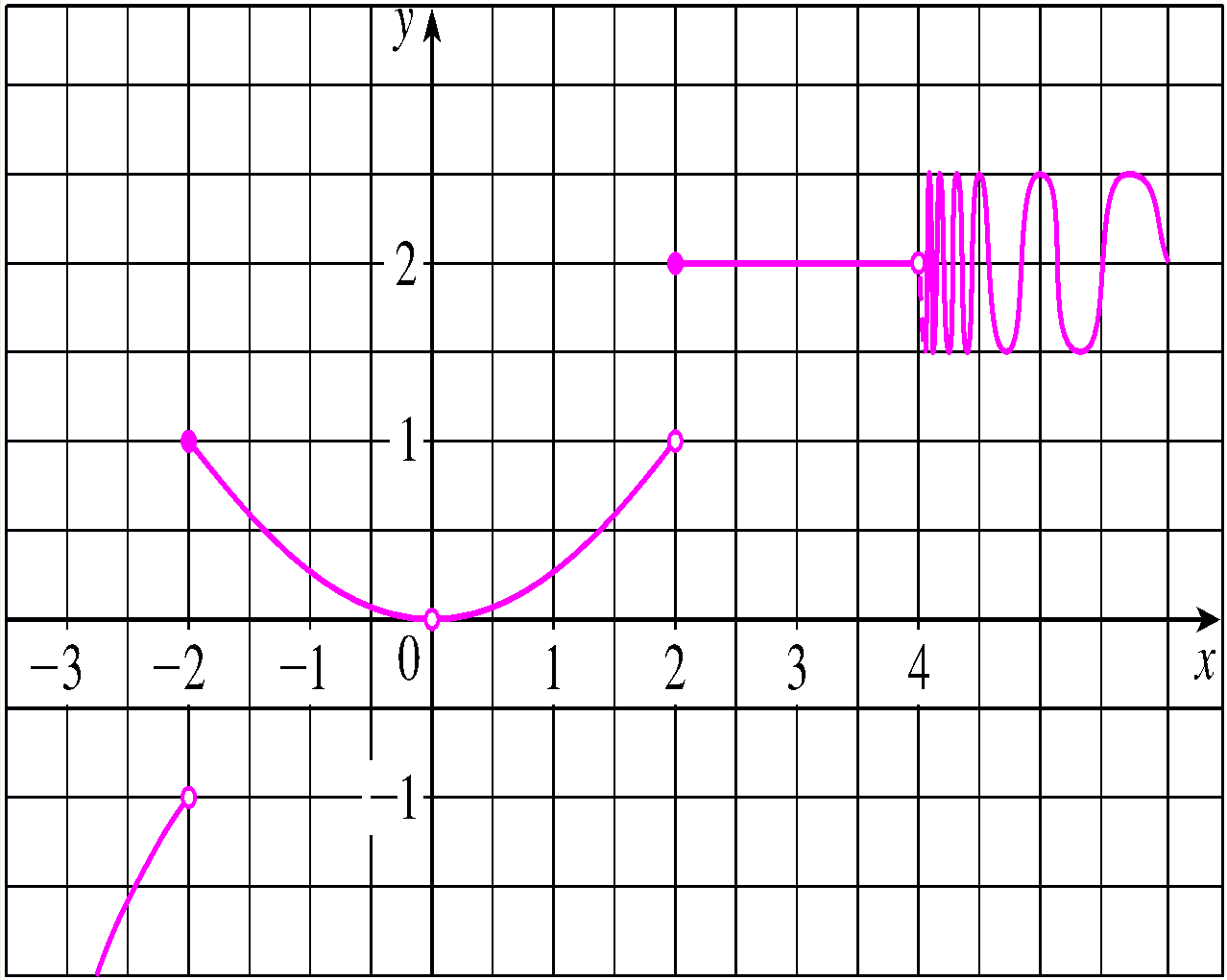For the function $f$ whose graph is given, state the value of the given quantity, if it exists. If it does not exist, enter "n" below.

(a) $\displaystyle \lim_{x \to -2^-} f(x)$
(b) $\displaystyle \lim_{x \to -2^+} f(x)$
(c) $\displaystyle \lim_{x \to -2} f(x)$
(d) $f(-2)$
(e) $\displaystyle \lim_{x \to 2^-} f(x)$
(f) $\displaystyle \lim_{x \to 2^+} f(x)$
(g) $\displaystyle \lim_{x \to 2} f(x)$
(h) $f(2)$
(i) $\displaystyle \lim_{x \to 4^+} f(x)$
(j) $\displaystyle \lim_{x \to 4^-} f(x)$
(k) $f(0)$
(l) $\displaystyle \lim_{x \to 0} f(x)$(a)
(b)
(c)
(d)
(e)
(f)
(g)
(h)
(i)
(j)
(k)
(l)

You can earn partial credit on this problem.